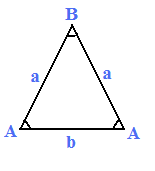## Isosceles Triangle Calculatorside a side b angle A angle B
 Area: Perimeter: Circumscribed Circle Radius: Inscribed Circle Radius: Height of a: Height of b: Angle Bisector of A: Angle Bisector of B: Median of a: Median of b:

In geometry, an isosceles triangle is a triangle that has two sides of equal length.

By the isosceles triangle theorem, the two angles opposite the equal sides are themselves equal, while if the third side is different then the third angle is different.

The isosceles calculator could gets area, perimeter, Circumscribed Circle Radius, Inscribed Circle Radius and so on.

### Isosceles Triangle Equations formual

 Perimeter 2 × a + b Area (K) K2 = b2 * (4 × a2 - b2) / 16 Angle Bisector of Side b ( tb ) tb2 = 4 × a2 - b2 / 2 Media of Side a ( ma ) ma2 = (2 * b2 + a2) / 4 Angle Bisector of Side a( tb ) ta2 = b2 × a × (2a + b) / (a + b)2 Media of Side b ( mb ) mb2 = (4 * a2 - b2) / 4 Inscribed Circle Radius (r) r2 = b2 × (2a - b) / (4 × (2a + b)) Circumscribed Circle Radius a / ( 2 * sin (A)) or b / ( 2 * sin (B))
Thinkcalculator.com provides you helpful and handy calculator resources.